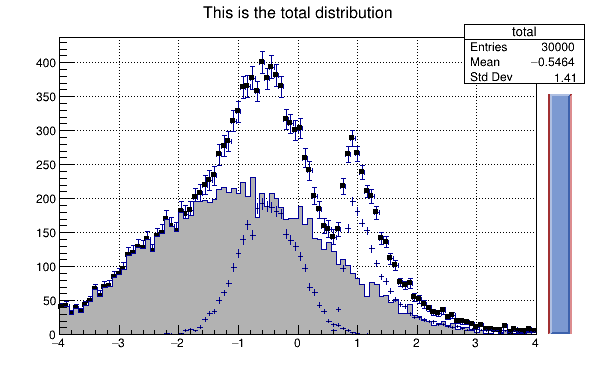# Hsumanim¶

This script is a slightly modified version of hsum.C. When run in batch mode, it produces an animated gif file.

Author: Rene Brun, Valeriy Onuchin
This notebook tutorial was automatically generated with ROOTBOOK-izer from the macro found in the ROOT repository on Monday, February 17, 2020 at 01:59 AM.

In :
auto c1 = new TCanvas("c1","The HSUM example",200,10,600,400);
c1->SetGrid();

gBenchmark->Start("hsum");


Create some histograms.

In :
auto total  = new TH1F("total","This is the total distribution",100,-4,4);
auto main   = new TH1F("main","Main contributor",100,-4,4);
auto s1     = new TH1F("s1","This is the first signal",100,-4,4);
auto s2     = new TH1F("s2","This is the second signal",100,-4,4);
total->Sumw2();   // this makes sure that the sum of squares of weights will be stored
total->SetMarkerStyle(21);
total->SetMarkerSize(0.7);
main->SetFillColor(16);
s1->SetFillColor(42);
s2->SetFillColor(46);
TSlider *slider = 0;


Fill histograms randomly

In :
gRandom->SetSeed();
const Int_t kUPDATE = 500;
Float_t xs1, xs2, xmain;
Int_t gifcnt = 0;
for ( Int_t i=0; i<10000; i++) {
xmain = gRandom->Gaus(-1,1.5);
xs1   = gRandom->Gaus(-0.5,0.5);
xs2   = gRandom->Landau(1,0.15);
main->Fill(xmain);
s1->Fill(xs1,0.3);
s2->Fill(xs2,0.2);
total->Fill(xmain);
total->Fill(xs1,0.3);
total->Fill(xs2,0.2);
if (i && (i%kUPDATE) == 0) {
if (i == kUPDATE) {
total->Draw("e1p");
main->Draw("same");
s1->Draw("same");
s2->Draw("same");
c1->Update();
slider = new TSlider("slider","test",4.2,0,4.6,total->GetMaximum(),38);
slider->SetFillColor(46);
}
if (slider) slider->SetRange(0,Float_t(i)/10000.);
c1->Modified();
c1->Update();
if (gROOT->IsBatch()) {
c1->Print("hsumanim.gif+");
printf("i = %d\n", i);
} else {
if (gSystem->ProcessEvents())
break;
}
}
}
slider->SetRange(0,1);
total->Draw("sameaxis"); // to redraw axis hidden by the fill area
c1->Modified();

i = 500
i = 1000
i = 1500
i = 2000
i = 2500
i = 3000
i = 3500
i = 4000
i = 4500
i = 5000
i = 5500
i = 6000
i = 6500
i = 7000
i = 7500
i = 8000
i = 8500
i = 9000
i = 9500


Make infinite animation by adding "++" to the file name

In :
if (gROOT->IsBatch()) c1->Print("hsumanim.gif++");


You can view the animated file hsumanim.gif with netscape/ie or mozilla

In :
gBenchmark->Show("hsum");

hsum      : Real Time =   1.36 seconds Cpu Time =   1.15 seconds


Draw all canvases

In :
gROOT->GetListOfCanvases()->Draw()